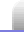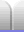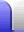# Pythagoras' Theorem

### Pythagoras' Theorem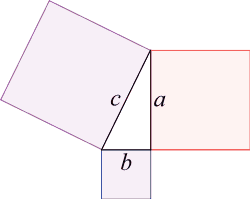Pythagoras' theorem states that the square of the hypotenuse will equal the sum of the squares of the other two sides. (It doesn't matter which way around a and b are.) a2 + b2 = c2 Two easy to remember right triangles which fulfill this condition with whole numbers are the 3, 4, 5 triangle and the 5, 12, 13 triangle. 32 + 42 = 52 9 + 16 = 25 52 + 122 = 132 25 + 144 = 169 These three numbers are together called Pythagorean triples.

### Proving Pythagoras' theorem

 One way of proving Pythagoras' theorem is by algebra. We can arrange four triangle so that they bound a square area (light blue). We know it's a square because the angles in a triangle add to 180° and so do angles in a straight line. The area of a triangle is given by 1/2 base x height, and the area of a square is given by (length)2. So the area of the whole arrangement in the diagram will be given by the area of 4 triangles plus the large blue square. 4 x (1/2 a x b ) + c2 But the arrangement is a square - there is a right angle at each corner and each side has a length of a + b. So the total area can also be given by: ( a + b )2 Of course, these areas are the same, so we equate the two then simplify. ( a + b )2 = 4( 1/2 ab ) + c2 ( a + b )( a + b ) = 4/2 ab + c2 a2 + ab + ba + b2 = 2ab + c2 a2 + 2ab + b2 = 2ab + c2 a2 + b2 = c2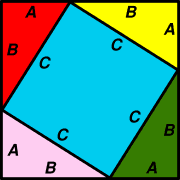It's also possible to prove by rearrangement.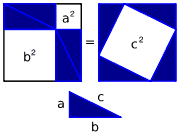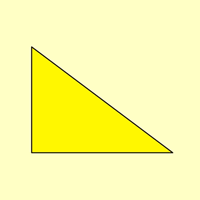What happens if you double the size of one of the examples given, say 3, 4, 5 becomes 6, 8, 10? Will the triangle still be a right angle triangle? Do the numbers still work out for Pythagoras' theorem?

For information see the Pythagoreas Triples page.Home Astronomy Chemistry Electronics & Computers Mathematics Physics Field Trips Turn on javascript for email link